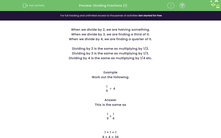# Dividing Fractions (1)

In this worksheet, students divide unit fractions by whole numbers.Key stage:  KS 2

Curriculum topic:   Number: Fractions, Decimals and Percentages

Curriculum subtopic:   Divide Proper Fractions

Difficulty level:#### Worksheet Overview

When we divide by 2, we are halving something.

When we divide by 3, we are finding a third of it.

When we divide by 4, we are finding a quarter of it.

Dividing by 2 is the same as multiplying by 1/2.

Dividing by 3 is the same as multiplying by 1/3.

Dividing by 4 is the same as multiplying by 1/4 etc.

Example

Work out the following.

 1 ÷ 4 9

This is the same as

 1 × 1 9 4

1 × 1 = 1

9 × 4 = 36

 1 36

which we write as   1/36.

Want a bit more help with this before you begin? Why not watch this short video?

### What is EdPlace?

We're your National Curriculum aligned online education content provider helping each child succeed in English, maths and science from year 1 to GCSE. With an EdPlace account you’ll be able to track and measure progress, helping each child achieve their best. We build confidence and attainment by personalising each child’s learning at a level that suits them.

Get started